# How do Long Short Term Memory (LSTM) and Gated Recurrent Unit (GRU) work in Deep Learning?

• Tanesh Balodi
• Dec 10, 2019
• Deep LearningLSTM networks were designed for long term dependencies, therefore the idea which makes it different from other neural network is that it is able to remember information for a long span of time without learning, again and again, making this whole process simpler and faster. This type of recurrent neural network includes an inbuilt memory system for storing information.

Introduced in the year 2014, GRU is broadly known as a gated recurrent unit and is a very effective technique under recurrent neural network, if we say that GRU is an updated and better version of LSTM we would not be that wrong, GRU tends to work faster in comparison to LSTM as it does not contain any memory unit, but in many models, it is tested that both GRU and LSTM has provided the same results. Further, in the blog, we will be discussing it’s working in comparison with LSTM and lots more.

## Topics Covered

1. Why do we use LSTM and GRU?

2. Working and Comparison of LSTM and GRU

3. Applications of LSTM and GRU

4. Conclusion

## Why do we use GRU and LSTM?

Generally, both LSTM and GRU are used with the intuition to solve the vanishing gradient issue, LSTM has a complex design when compared to GRU which is much simpler. Both LSTM and GRU have gates, and the whole working is dependent upon these gates, however, GRU has simplified gates which makes it easier to understand.

As we have discussed above, GRU is faster than LSTM, apart from its speed, it is able to handle the vanishing gradient problem very well. For those who are not aware of the term, the vanishing gradient problem is a loss of information that occurs while training our model with the help of gradient descent or other techniques where we tend to find loss while back-propagating in a network to extract out valuable arbitrary functions or information, while we back-propagate in our network, our gradients become smaller which means we cannot extract the information with full potential and this will cause a poor model accuracy. Let's quickly move towards the working of LSTM and GRU.

## Working and Comparison of LSTM and GRU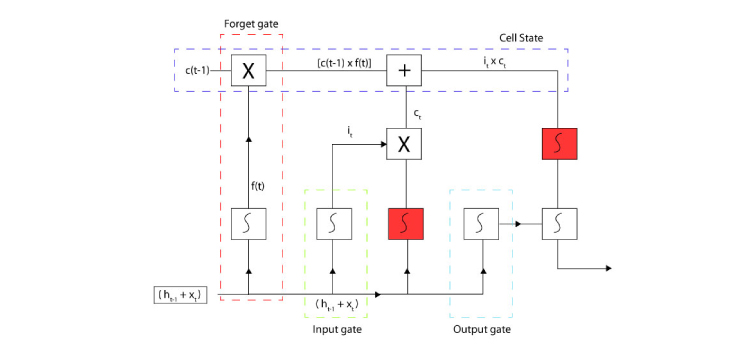Working model of Long Short Term Memory (LSTM)

Above structure is of LSTM, it has five main components:-

1. Forget Gate

2. Input Gate

3. Cell State

4. Output Gate

5. Hidden State Output

### 1. Forget Gate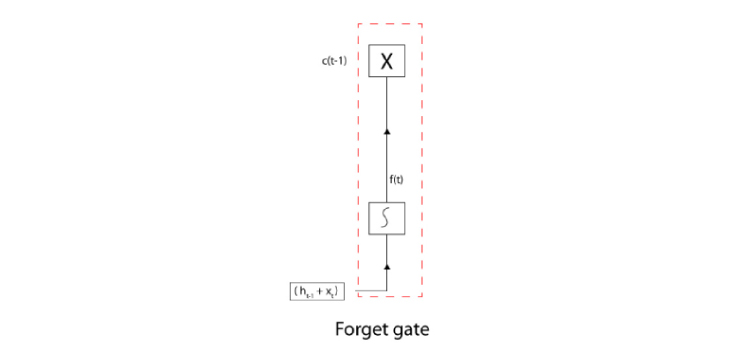Structure of the Forget Gate of LSTM

This gate is sorting out the relevant and irrelevant information and pushing forward, only the relevant information towards the cell state ( ht-1 + xt) is forwarded to forget gate where ht-1 is the previous hidden state and xt is current input, the addition of both is processed under sigmoid function which will convert the output value in the range 0 to 1. So how does it sorts out irrelevant information and relevant information? It does not push forward any value which is closer to zero as it is irrelevant and every value which is closer to one is pushed forward towards the cell state of the LSTM structure. Therefore at cell state, the equation becomes (ct-1) * (ft). Here (ct-1)  is the previous cell state.

### 2. Input Gate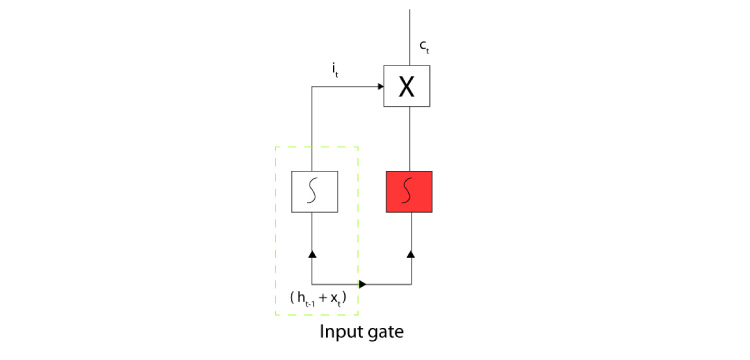Structure of the Input Gate of LSTM

This gate is processing the ( ht-1 + xt) and giving out new input using the activation function which is usually Sigmoid activation function, again in the range of 0 to 1, not ignoring any information like forget gate. New input is generated which is known as the input gate input (it), while on the other hand  (ct) is being processed under the Tanh activation function which generates the output in the range of -1 to 1. This new input information is multiplied further with the candidate input (ct) which turns the input into  (ct) * (it). This information is also passed to the cell state.

### 3. Cell StateStructure of the Cell State of LSTM

The upcoming information from the forget gate i.e  (ct) * (ft) added with the information coming from input gate i.e  (ct) * (it) which makes the whole equation to be

Cell state =   (ct-1) * (ft)     +  (ct) * (it)

Therefore, from the equation and the step by step guide, you can notice that cell state act as a memory unit of the LSTM structure as it contains all the relevant information.

### 4. Output Gate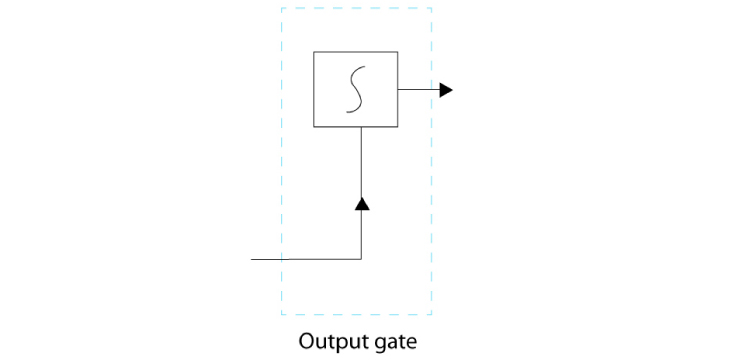Processing of an Output Gate of LSTM

Output gate processes ( ht-1 + xt) under the Sigmoid Activation function which squeezes the information in the range of 0 to 1, is further multiplied with the cell state information. This cell state information is also processed under the Tanh Activation function, all the multiplication done is Hadamard product which is nothing but element-wise multiplication. Cell state information is multiplied with the output information (ot).

### 5. Hidden state output

Hidden state output is the multiplication of cell state information and output information

ht =     [  (ct-1) * (ft)     +  (ct) * (it)   ] *(tanh) *(ot)

Presently experiment over activation functions such as Softmax, ReLU, and leaky ReLU activation function presented more optimum results, we have seen in many models that these activations functions help in avoiding vanishing gradient problem.

Now let’s see how GRU works:-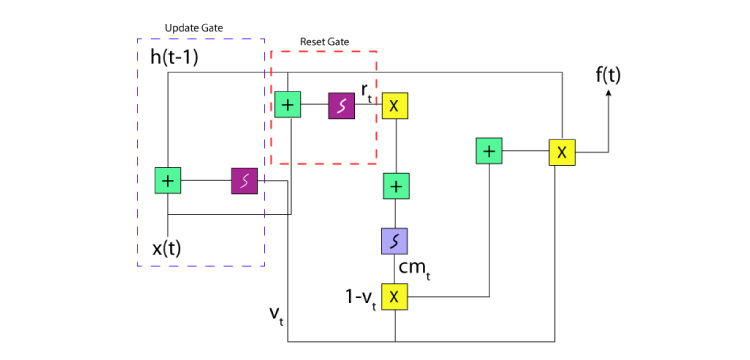The working architecture of Gated Recurrent Unit(GRU)

The main problem with the recurrent neural network was that it could not remember the older information no matter whether it was relevant or irrelevant, which itself was against the idea of RNN, but GRU has been proved to remember every piece of information, even if that information is turned out to be irrelevant, so this technique holds the very idea of recurrent neural network.GRU also uses gates like LSTM but not too many, the gates used in GRU are update gates and reset gates, we will broadly discuss them.

The main components of GRU are:-

###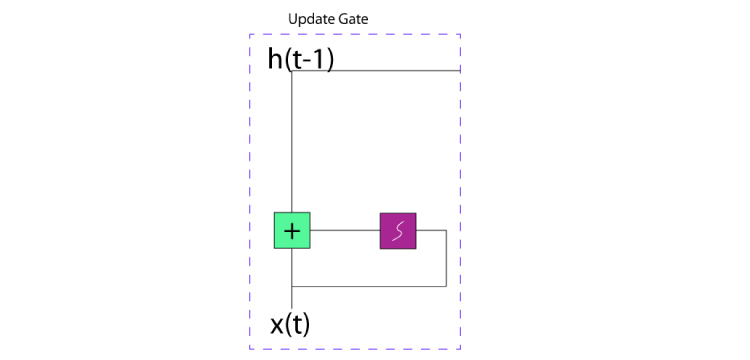Presentation of an Update Gate of GRU

At this gate, the network learns how much past information to push forward, here the input (xt)  along with its weight (wt) are multiplied together which are further added with the multiplication of previous hidden state (ht-1) along with its weight (wh). Further, this value is processed under the sigmoid function to compress the value in the range of 0 to 1. Hence the update gate helps in curing vanishing gradient issues.

ut =  sigmoid  [ (xt)  *  (wt)   + (ht-1)  * (wh) ]

### 2. Reset Gate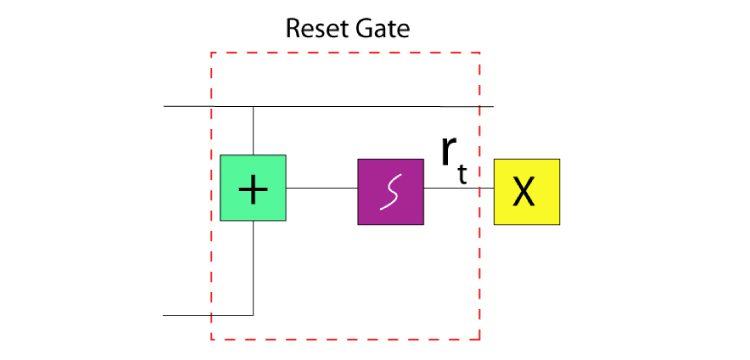Structure of the Reset Gate of GRU

Reset gate is almost the same as the Forget gate of LSTM, as it sorts the irrelevant data and tells the model to forget this data and move forward without it. However, the formula is almost the same as the update gate and only differs at weights and functionality.

rt = sigmoid [ (xt)  * (wr)  + (ht-1)  * (whr) ]

If we observe closely, it functions the exact opposite of what the Update Gate does. Here, the Sigmoid Function converts the value in the range that lies between 0 to 1 and the value closer to zero will not be used further while the value ranging closer to 1 will be processed forward.

### 3. Current Memory Content

Let's consider an example of a movie review “Chhichhore’ has a relevant message on the inherent attitude towards academic success and failure that will connect with many youngsters and parents of today. It tells you that the journey is far more important than the destination and that losing is as critical a life lesson as winning. The film scores high on many accounts and is certainly worth watching.

As we can see the last line depicts the result or conclusion of the review, so our neural network will learn with the help of the reset gate to ignore all other information written above, current memory content uses reset gate for the functioning like sentiment analysis. Let’s see it’s equation:-

cmt = tanh (wxt +  rt x yht-1)

Here, xt is multiplied with its weight w and added to the Hadamard product of previously hidden state multiple of its weight. Here, the Tanh function is used to make the values to fit in the range that lies between -1 to 1.

### 4. Final Memory at Current time state

Let's suppose that while doing sentiment analysis of a movie review, we found the best information at the very first line itself and all other information in the text is of no use, then our model must be able to sort the sentiment out from the first line and ignore other text. It basically carries the current state input which needs the Update gate, hence the Update gate is an essential prerequisite for this final stage. Let's see its equation:-

ft      =  ut x (ht-1)  + (1-ut) h't

Here, ‘X’ shows the Hadamard product of the Update gate and current information which is added to the Hadamard product of (1-ut) and h't where h't show the information or content that is needed to be collected from current information unit.

## Applications of LSTM and GRU

1. Speech Recognition

2. Speech Synthesis

3. Sentiment Analysis

4. Stock Price Prediction

5. Machine Comprehension

## Conclusion

In the series of deep learning and machine learning blogs, I came up with the core concept and functioning of LSTM and GRU. In this blog, we learned about LSTM and GRU with their working, I hope you got the fundamentals which will lead you forward in recurrent neural networks, these two methods will be helpful to you in understanding natural language processing, try experimenting with these methods. For more blogs in Analytics and new technologies do read Analytics Steps.

0%